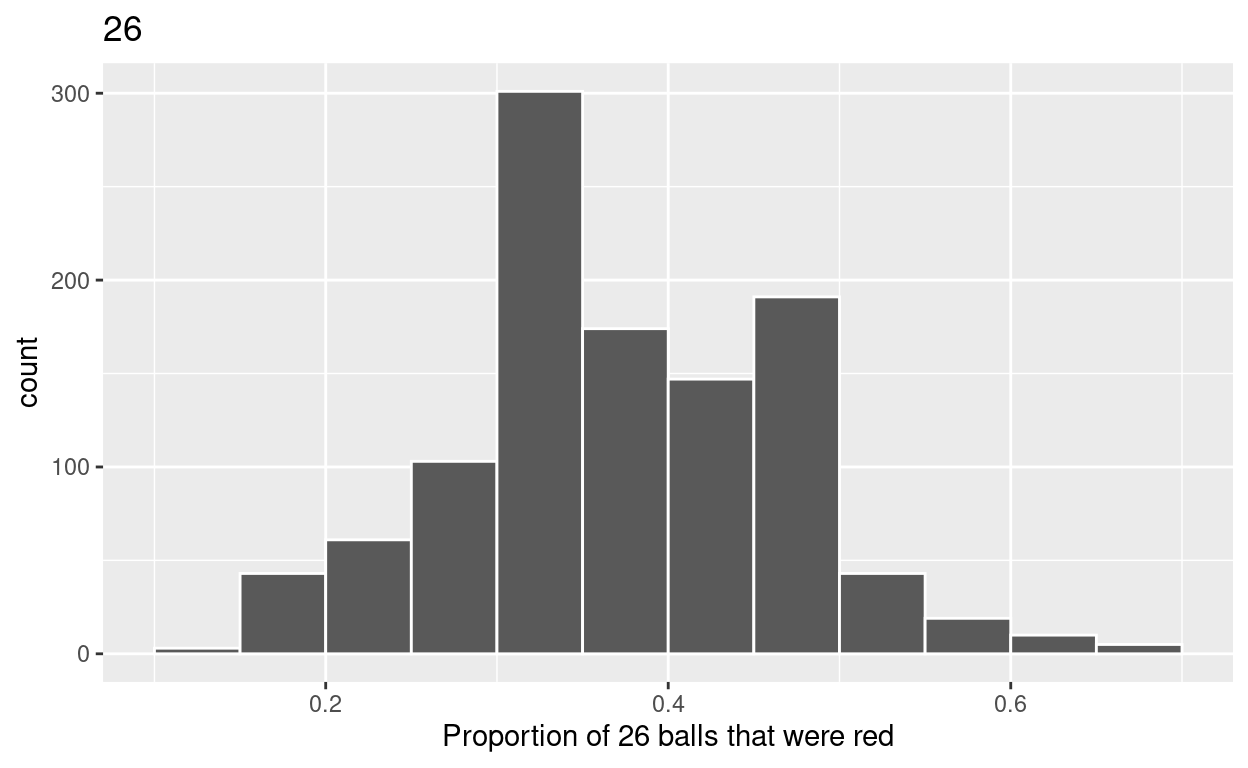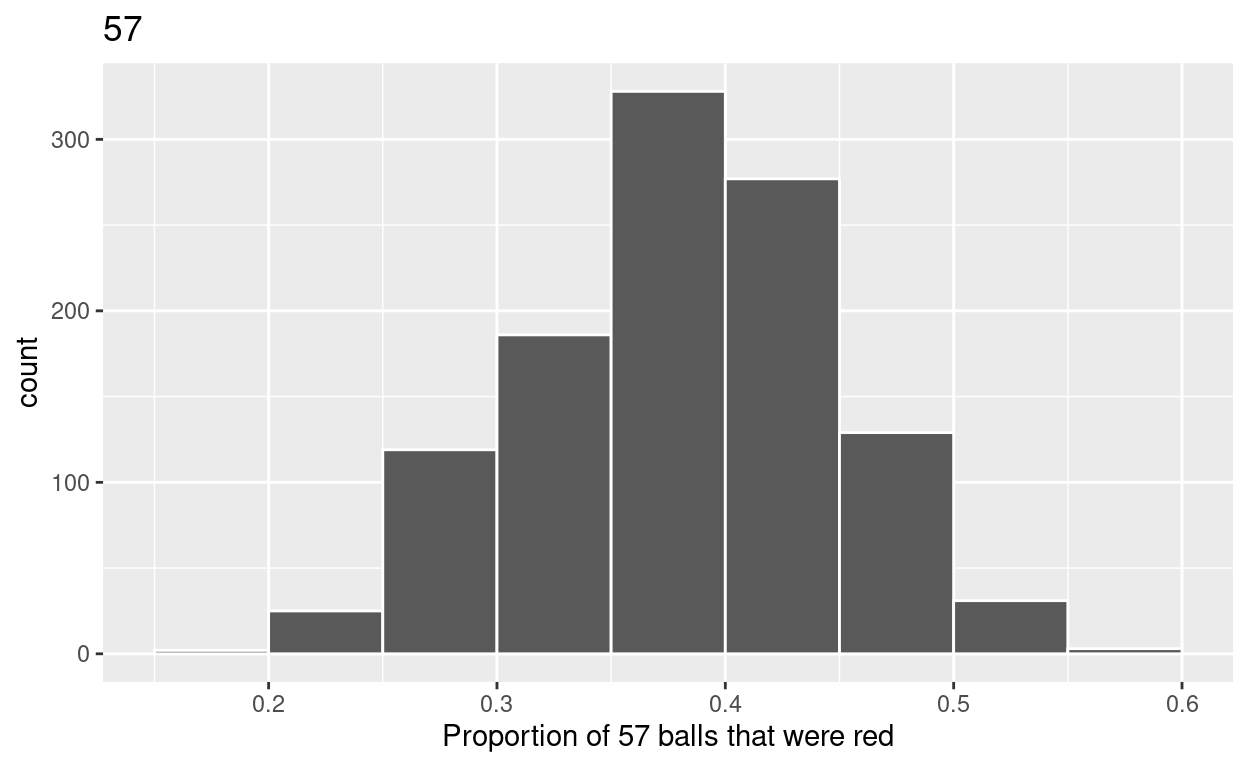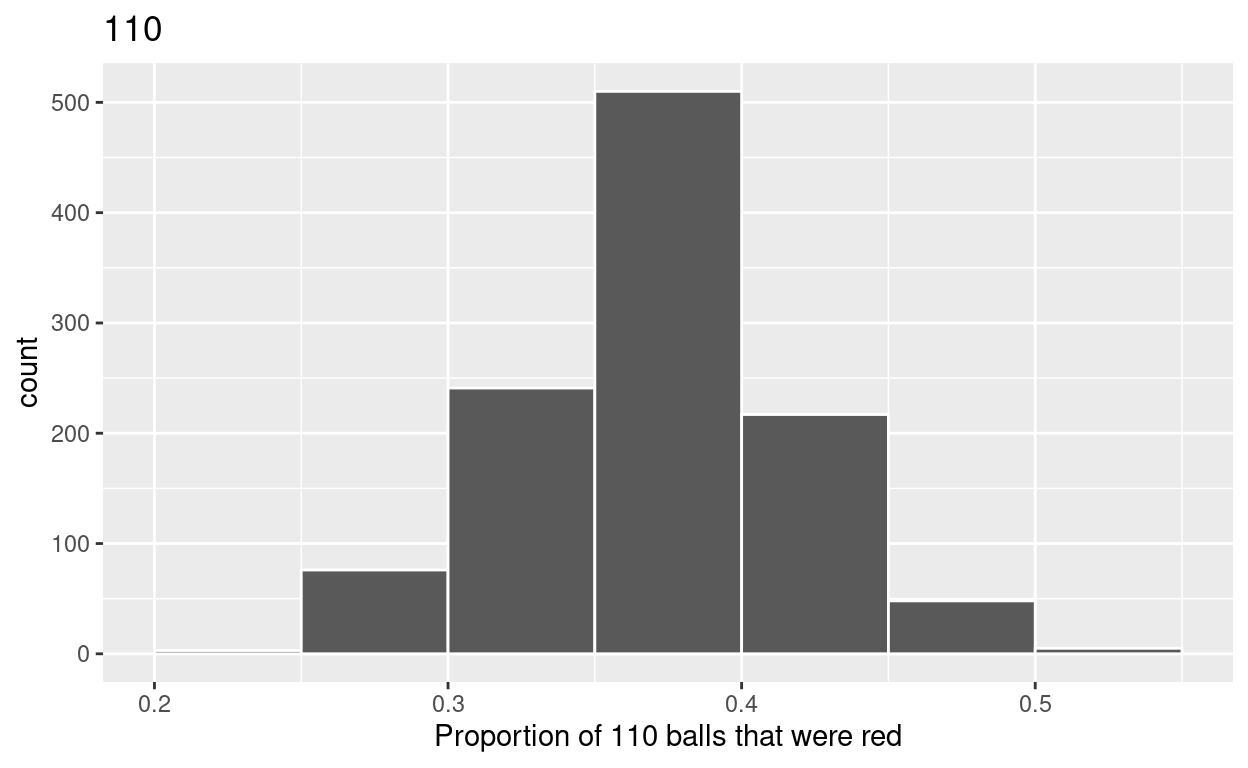# Sampling

Based on Chapter 7 of ModernDive. Code for Quiz 11.

1. Load the R packages we will use.
``````library(tidyverse)
library(moderndive)
``````
1. Quiz questions
• Replace all the instances of ‘SEE QUIZ’. These are inputs from your moodle quiz.

• Replace all the instances of ‘???’. These are answers on your moodle quiz.

• Run all the individual code chunks to make sure the answers in this file correspond with your quiz answers

• After you check all your code chunks run then you can knit it. It won’t knit until the ??? are replaced

• The quiz assumes that you have watched the videos and worked through the examples in Chapter 7 of ModernDive

# Question

7.2.4 in Modern Dive with different sample sizes and repetitions

• Make sure you have installed and loaded the `tidyverse` and the `moderndive` packages

• Fill in the blanks

• Put the command you use in the Rchunks in your Rmd file for this quiz.

**Modify the code for comparing different sample sizes from the `virtual bowl`

Segment 1: sample size = 26**

1.a) Take 1100 samples of size of 26 instead of 1000 replicates of size 25 from the `bowl` dataset. Assign the output to virtual_samples_26

``````virtual_samples_26  <- bowl  %>%
rep_sample_n(size = 26, reps = 1100)
``````

1.b) Compute resulting `1100` replicates of proportion red

• start with `virtual_samples_26` THEN

• `group_by` replicate THEN

• create variable `red` equal to the sum of all the red balls

• create variable `prop_red equal` to variable red / `26`

• Assign the output to `virtual_prop_red_26`

``````virtual_prop_red_26 <- virtual_samples_26 %>%
group_by(replicate) %>%
summarize(red = sum(color == "red")) %>%
mutate(prop_red = red / 26)
``````

1.1.c) Plot distribution of virtual_prop_red_26 via a histogram use labs to

• label x axis = “Proportion of 26 balls that were red”

• create title = “26”

``````ggplot(virtual_prop_red_26, aes(x = prop_red)) +
geom_histogram(binwidth = 0.05, boundary = 0.4, color = "white") +
labs(x = "Proportion of 26 balls that were red", title = "26")
``````Segment 2: sample size = 57

2.a) Take 1100 samples of size of 57 instead of 1000 replicates of size 50. Assign the output to virtual_samples_57

``````virtual_samples_57  <- bowl  %>%
rep_sample_n(size = 57, reps = 1100)
``````

2.b) Compute resulting 1120 replicates of proportion red

• group_by replicate THEN

• create variable red equal to the sum of all the red balls

• create variable prop_red equal to variable red / 57

• Assign the output to virtual_prop_red_57

``````virtual_prop_red_57 <- virtual_samples_57 %>%
group_by(replicate) %>%
summarize(red = sum(color == "red")) %>%
mutate(prop_red = red / 57)
``````

2.c) Plot distribution of virtual_prop_red_57 via a histogram use labs to

• label x axis = “Proportion of 57 balls that were red”

• create title = “57”

``````ggplot(virtual_prop_red_57, aes(x = prop_red)) +
geom_histogram(binwidth = 0.05, boundary = 0.4, color = "white") +
labs(x = "Proportion of 57 balls that were red", title = "57")
``````Segment 3: sample size = 110

3.a) Take 1100 samples of size of 110 instead of 1000 replicates of size 50. Assign the output to virtual_samples_110

``````virtual_samples_110  <- bowl  %>%
rep_sample_n(size = 110, reps = 1100)
``````

3.b) Compute resulting 1100 replicates of proportion red

• group_by replicate THEN

• create variable red equal to the sum of all the red balls

• create variable prop_red equal to variable red / 110

• Assign the output to virtual_prop_red_110

``````virtual_prop_red_110 <- virtual_samples_110 %>%
group_by(replicate) %>%
summarize(red = sum(color == "red")) %>%
mutate(prop_red = red / 110)
``````

3.c) Plot distribution of virtual_prop_red_110 via a histogram use labs to

• label x axis = “Proportion of 110 balls that were red”

• create title = “110”

``````ggplot(virtual_prop_red_110, aes(x = prop_red)) +
geom_histogram(binwidth = 0.05, boundary = 0.4, color = "white") +
labs(x = "Proportion of 110 balls that were red", title = "110")
````````````ggsave(filename = "preview.png",
path = here::here("_posts", "2022-04-19-sampling"))
``````

Calculate the standard deviations for your three sets of 1100 values of `prop_red` using the `standard deviation`

n = 26

``````virtual_prop_red_26 %>%
summarize(sd = sd(prop_red))
``````
``````# A tibble: 1 × 1
sd
<dbl>
1 0.0972``````

n = 57

``````virtual_prop_red_57 %>%
summarize(sd = sd(prop_red))
``````
``````# A tibble: 1 × 1
sd
<dbl>
1 0.0650``````

n = 110

``````virtual_prop_red_110 %>%
summarize(sd = sd(prop_red))
``````
``````# A tibble: 1 × 1
sd
<dbl>
1 0.0463``````